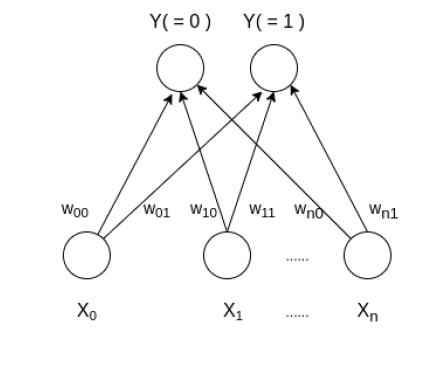Related Articles

# Learning Vector Quantization

• Difficulty Level : Expert
• Last Updated : 10 Jul, 2020

Learning Vector Quantization ( or LVQ ) is a type of Artificial Neural Network which also inspired by biological models of neural systems. It is based on prototype supervised learning classification algorithm and trained its network through a competitive learning algorithm similar to Self Organizing Map. It can also deal with the multiclass classification problem. LVQ has two layers, one is the Input layer and the other one is the Output layer. The architecture of the Learning Vector Quantization with the number of classes in an input data and n number of input features for any sample is given below:### How Learning Vector Quantization works?

Let say an input data of size ( m, n ) where m is number of training example and n is the number of features in each example and a label vector of size ( m, 1 ). First, it initializes the weights of size ( n, c ) from the first c number of training samples with different labels and should be discarded from all training samples. Here, c is the number of classes. Then iterate over the remaining input data, for each training example, it updates the winning vector ( weight vector with the shortest distance ( e.g Euclidean distance ) from training example ). Weight updation rule is given by :

`wij = wij(old) - alpha(t) * (xik - wij(old))`

where alpha is a learning rate at time t, j denotes the winning vector, i denotes the ith feature of training example and k denotes the kth training example from the input data. After training the LVQ network, trained weights are used for classifying new examples. A new example labeled with the class of winning vector.

### Algorithm

Steps involved are :

• Weight initialization
• For 1 to N number of epochs
• Select a training example
• Compute the winning vector
• Update the winning vector
• Repeat steps 3, 4, 5 for all training example.
• Classify test sample

Below is the implementation.

 `import` `math`` ` ` ` `class` `LVQ :``     ` `    ``# Function here computes the winning vector``    ``# by Euclidean distance``    ``def` `winner( ``self``, weights, sample ) :``         ` `        ``D0 ``=` `0``        ``D1 ``=` `0``         ` `        ``for` `i  ``in` `range``( ``len``( sample ) ) :``            ``D0 ``=` `D0 ``+` `math.``pow``( ( sample[i] ``-` `weights[``0``][i] ), ``2` `)``            ``D1 ``=` `D1 ``+` `math.``pow``( ( sample[i] ``-` `weights[``1``][i] ), ``2` `)``             ` `            ``if` `D0 > D1 :``                ``return` `0``            ``else` `: ``                ``return` `1`` ` `    ``# Function here updates the winning vector     ``    ``def` `update( ``self``, weights, sample, J, alpha ) :``        ``for` `i ``in` `range``(``len``(weights)) :``            ``weights[J][i] ``=` `weights[J][i] ``+` `alpha ``*` `( sample[i] ``-` `weights[J][i] ) `` ` `# Driver code``def` `main() :`` ` `    ``# Training Samples ( m, n ) with their class vector``    ``X ``=`  `[[ ``0``, ``0``, ``1``, ``1` `],  [ ``1``, ``0``, ``0``, ``0` `], ``          ``[ ``0``, ``0``, ``0``, ``1` `], [ ``0``, ``1``, ``1``, ``0` `],``          ``[ ``1``, ``1``, ``0``, ``0` `], [ ``1``, ``1``, ``1``, ``0` `],] `` ` `    ``Y ``=` `[ ``0``, ``1``, ``0``, ``1``, ``1``, ``1` `]``    ``m, n ``=` `len``( X ), ``len``( X[``0``] )``     ` `    ``# weight initialization ( n, c )``    ``weights ``=` `[]``    ``weights.append( X.pop( ``0` `) )``    ``weights.append( X.pop( ``1` `) )`` ` `    ``# Samples used in weight initialization will``    ``# not use in training``    ``m ``=` `m ``-` `2``     ` `    ``# training``    ``ob ``=` `LVQ()``    ``epochs ``=` `3``    ``alpha ``=` `0.1``     ` `    ``for` `i ``in` `range``( epochs ) :``        ``for` `j ``in` `range``( m ) :``             ` `            ``# Sample selection``            ``T ``=` `X[j]``             ` `            ``# Compute winner``            ``J ``=` `ob.winner( weights, T )``         ` `            ``# Update weights``            ``ob.update( weights, T, J, alpha )``             ` `    ``# classify new input sample``    ``T ``=` `[ ``0``, ``0``, ``1``, ``0` `]``    ``J ``=` `ob.winner( weights, T )``    ``print``( ``"Sample T belongs to class : "``, J )``    ``print``( ``"Trained weights : "``, weights )``     ` `if` `__name__ ``=``=` `"__main__"``:``    ``main()`
Output:

Sample T belongs to class : 0
Trained weights : [[0.3660931, 0.38165410000000005, 1, 1], [0.33661, 0.34390000000000004, 0, 1]]

Attention geek! Strengthen your foundations with the Python Programming Foundation Course and learn the basics.

To begin with, your interview preparations Enhance your Data Structures concepts with the Python DS Course. And to begin with your Machine Learning Journey, join the Machine Learning – Basic Level Course

My Personal Notes arrow_drop_up